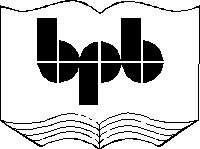4

Lang library

What is a library?

A programming language basically offers an environment encompassing conditional statements such as, the, if and for constructs, loops, arithmetic and logical operators etc. But, what really separates one programming language from another is its ability to enhance the productivity of the programmer. This is achieved by distributing free libraries, functions and utilities with the programming language. In this case, a large number of very useful functions have been provided for free.

In the case of WMLScript, a large number of functions have been provided. But, they have not been made available directly. Instead, they have been  distributed as part of the library. Functions are classified  into 6 libraries based on their functionality and purpose. The names of the libraries have been standardised for future expansion and upward scalability. Some programming languages like C have also provided libraries of functions, but they have not standardised the names. This chapter, thus, delves into the lang library .

The first function that we use here is float.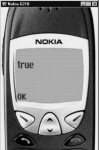Screen 4. 1

aaa.wmls

extern function abc()

{

var i;

i = Lang.float();

if (i)

else

}

Float returns a value of true or false. If the WMLScript user agent supports numbers i.e. floating  point numbers, then the float function will return a true. In our case, the user agent viz. Nokia, supports numbers with decimal places.

Numbers with decimal places generally create problems. If you take 10 and divide it by 3, the answer is 3.333333. The number of decimal places can go on indefinitely. No computer or microprocessor understands how to work with numbers with decimal places. They are either simulated in software or there are special microprocessors made for this purpose. Like the earlier Intel chips, the 286  never understood numbers with decimal places. Either you had to buy a special chip called a numeric coprocessor, or you were required  to simulate it in software.

If you are performing calculations like mortgage payments, insurance payments etc., you obviously need numbers with decimal places. You don't have a choice in this matter. Now, WMLScript has to work with numbers that have decimal places. These numbers require more place than numbers without decimal places. If your user agent does not support it ,  you have to abort the idea of using them.

When you deal with variables, all variables have to be stored in memory.  By default, if a variable deals with numbers, it is allocated 4 memory locations. In 4 memory locations, the upper limit is a number  which is 2 raised to 32, i.e. 4 billion. Unfortunately, this range has to be divided by half to cater to the positive and negative range of numbers.

The next function is maxInt. It is used to find out the largest number that is supported.

aaa.wmls

extern function abc()

{

var i,aa;

i = Lang.maxInt();

aa = String.format("%d ..",i);

i = Lang.minInt();

aa = String.format("%d ..",i);

}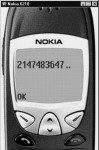Screen 4. 2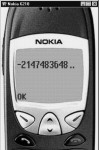Screen 4. 3

This function gives you the largest number that can be supported i.e. 2147483647 . If you add this number and its negative value, the result will be 4 billion, which is 2 raised to 32.

Every programming language has to be written using certain characters. All the allowable characters that can be used are collectively called the 'Character Set'. There is a body, which has allotted different numbers to different character sets.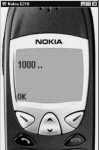Screen 4. 4

aaa.wmls

extern function abc()

{

var i,aa;

i = Lang.characterSet();

aa = String.format("%d ..",i);

}

In our user agent, the Lang.characterset returns a value of 1000. This represents the character set that we are using. According to the documentation, this number represents Latin which  is identical to English. If you use the ASCII Character Set, you will not be able to represent certain European characters.

aaa.wmls

extern function abc()

{

var i,aa;

i = 10.6;

aa = String.format("%d",i);

}

The next example shows that when you use String.format, you can use the %d format specifier. In String.format, you can use only two parameters :

· The first one is a format string that specifies as to how the variable is to be displayed.

· The second one is the variable.

In the example, the value of the variable i is 10.6. The format specifier %d means that a decimal is to be displayed.  %d we thought would remove the decimal point and give the final value as 10. But this does not happen here.  So, if you want to display a number that has decimal places, you have to use the format specifier of  %f.

aaa.wmls

extern function abc()

{

var i,aa;

i = 10.6;

aa = String.format("%f",i);

i = 10;

aa = String.format("%f",i);

}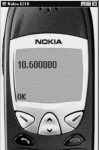Screen 4. 5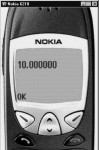Screen 4. 6

If you specify a value of 10 and use %f, the value that gets displayed will be 10.000000.  This is because the default number of decimal places is 6. Thus, whenever you want to display an integer, you can use %d; And, whenever you need to display a number with its decimal places, you can use %f.

Now, the next function that we introduce is max.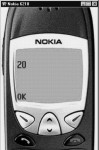Screen 4. 7

aaa.wmls

extern function abc()

{

var aa,i;

i = Lang.max(10,20);

aa = String.format("%d",i);

The function max accepts two numbers and returns the maximum of the two.  So, if you pass the numbers  10 and 20 to the function, it will return the value of 20. The data type of the returned value depends on the type of the larger number. If both numbers are equal, then the data type of the returned value is that of the first number. This does not sound very logical, however, we are left with  Hobson's choice of  mentioning it here, since the documentation talks about it.

Here, we submit another example.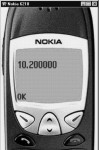Screen 4. 8

aaa.wmls

extern function abc()

{

var aa,i;

i = Lang.max(10,10.2);

aa = String.format("%f",i);

}

WMLScript does not truncate 10.2 to 10. Instead, it converts the 10 into 10.0 while changing it to float. Hence, the Lang.max function returns the value 10.2. The number will always be converted to the next higher level i.e. from an integer to a float. Internally, all numbers are converted to floating-point numbers.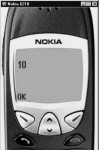Screen 4. 9

aaa.wmls

extern function abc()

{

var aa,i;

i = Lang.min(10,20);

aa = String.format("%d",i);

}

Complementary to the max function is a min function that returns the minimum of the two numbers which are supplied to it as parameters.Screen 4. 10

aaa.wmls

extern function abc()

{

var aa,i;

i = pqr(30,10);

aa = String.format("%d",i);

}

function pqr(y,z)

{

if (y = z)

return y;

else

return z;

}

The language really isn't required to provide functions like min and max, because they can  easily be written by a programmer, as shown above.

The next function that we shall explore is lang.abs.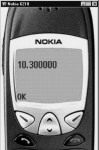Screen 4. 11

aaa.wmls

extern function abc()

{

var aa,i;

i = Lang.abs(-10.3);

aa = String.format("%f",i);

}

The Lang.abs function returns the absolute value of the number that is passed, as a parameter. If the number has a negative value, it gets converted to a positive value, whereas, if it is a positive value, it remains unchanged.

You can also replicate the functionality of the abs function by writing a function yourself. All that the function has to check is whether the parameter is greater than, or equal to 0. In case of either of them, the function has to return the same number; or else, it has to multiply it with -1 and then return the result.

The Lang.random function returns a random number. This function has been explained before.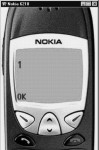Screen 4. 12

aaa.wmls

extern function abc()

{

var aa,i;

i = Lang.random(10);

aa = String.format("%d",i);

}

A significant point to be noted is that we have supplied a seed to the Lang.random function.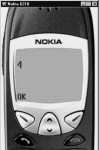Screen 4. 13

aaa.wmls

extern function abc()

{

var aa,i;

Lang.seed(5);

i = Lang.random(10);

aa = String.format("%d",i);

}

The seed that we supply to the random function ensures that it actually generates numbers randomly. Random returns an integer. If you give it an invalid value like "ABC" that can't be converted into a number, the function will generate an error and hence you see no output.

aaa.wmls

extern function abc()

{

var aa,i;

i = Lang.random("ABC");

aa = String.format("%d",i);

}

Both, Lang.exit and lang.abort, don't work as expected. Their output is not as intuitive as their names are. As per the documentation, Lang.exit stops the program and returns control back to the operating system. But practically, Lang.exit doesn't do so. Instead, it displays pqr and stops. So, in reality, there is no difference between the outputs generated by Lang.exit and  Lang.abort.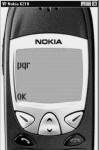Screen 4. 14

aaa.wmls

extern function abc()

{

var aa,i;

i = pqr();

aa = String.format("%d",i);

}

function pqr()

{

Lang.exit(30);

}Screen 4. 15

aaa.wmls

extern function abc()

{

var aa,i;

i = pqr();

aa = String.format("%d",i);

}

function pqr()

{

}

The next function that we take up is isInt.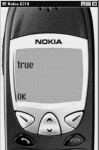Screen 4. 16

aaa.wmls

extern function abc()

{

var aa,i;

i = Lang.isInt(100);

if ( i )

else

}

The function isInt determines whether the parameter is of type int or not.  So, if you pass the value of 100 as a parameter to this function, it will obviously return true.  The function isInt doesn't perform any conversions. Instead, it gets this task done from a function called parseInt. If you pass the value 100.12 to lang.isInt, it will return true, since 100.12 can be converted to an integer. Similarly, parameters such as -78.90 and 89.8 will also return true. So, numbers with decimal places can be passed as parameters to this function without any problem.

But if you pass \$89.8 as a parameter, it will return a value of false, since  it  starts with a \$ sign. This happens because you are starting the parameter with a character. However, if you pass the value 89.8a, it will return true because the function will keep on reading the parameter till it spots an invalid character. Not only does the function parseInt  check whether the parameter has a positive or a negative sign, it also ensures that  the input is a sequence of numbers. As soon as it comes across a non-number, it stops accepting any further inputs. Therefore, the  value  accepted upto that particular point becomes the input. Thus, if the input value is 9+8, the answer is 9 and not 17.

Similar to the function isInt, there is a function  isFloat which uses the function parseFloat to check whether the value can be converted to a float or not..  The rules for this function are similar to those of parseInt.Screen 4. 17

aaa.wmls

extern function abc()

{

var aa,i;

i = Lang.isFloat(100);

if ( i )